Question

# The standard enthalpy change for the following reaction is 220 kJ at 298 K. CuCl2(s) -->Cu(s)...

The standard enthalpy change for the following reaction is 220 kJ at 298 K.

CuCl2(s) -->Cu(s) + Cl2(g)   ΔH° = 220 kJ

What is the standard enthalpy change for this reaction at 298 K?

 Cu(s) + Cl2(g) --> CuCl2(s)

The standard enthalpy change for the reaction is 220 kJ at 298 K.

CuCl2(s) -->Cu(s) + Cl2(g)   ΔH° = 220 kJ

given the reaction

Cu(s) + Cl2(g) --> CuCl2(s)

the above reaction is reverse of the standard reaction

so delta H will be same in magnitude and but the sign will be opposite

so

Cu(s) + Cl2(g) --> CuCl2(s)       ΔH° =    - 220 kJ (it is -ve value )

#### Earn Coins

Coins can be redeemed for fabulous gifts.

Similar Homework Help Questions
• ### The standard enthalpy change for the following reaction is 641 kJ at 298 K. MgCl2(s) Mg(s)...

The standard enthalpy change for the following reaction is 641 kJ at 298 K. MgCl2(s) Mg(s) + Cl2(g) ΔH° = 641 kJ What is the standard enthalpy change for this reaction at 298 K? Mg(s) + Cl2(g) MgCl2(s)

• ### The standard enthalpy change for the following reaction is -704 kJ at 298 K. Al(s) +...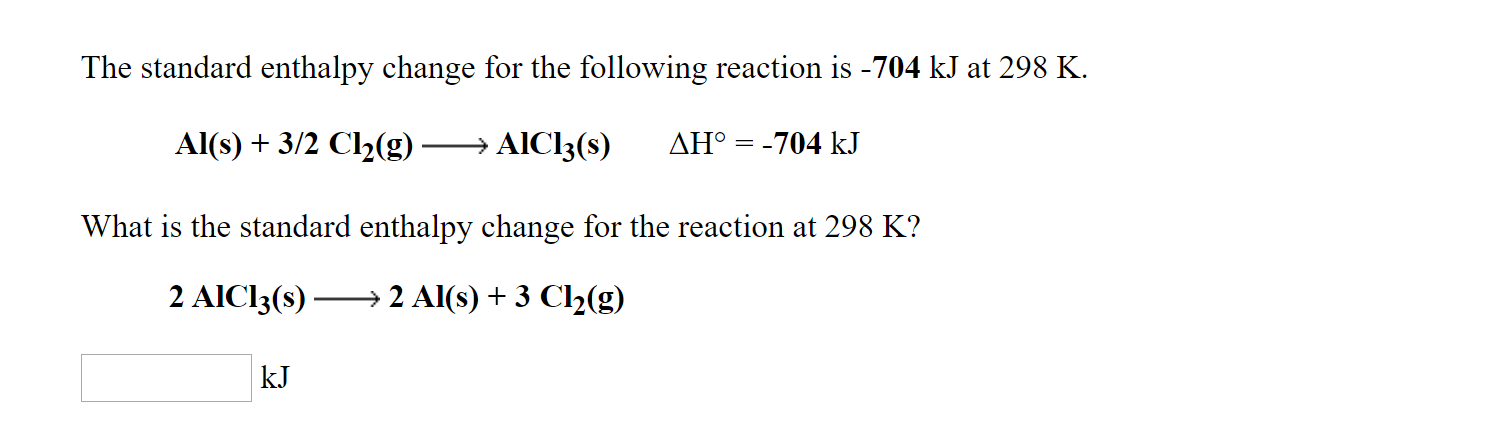The standard enthalpy change for the following reaction is -704 kJ at 298 K. Al(s) + 3/2 Cl2(g) — AICI3(8) AH° = -704 kJ What is the standard enthalpy change for the reaction at 298 K? 2 AICI3(s) — 2 Al(s) + 3 Cl2(9) kJ The standard enthalpy change for the following reaction is 851 kJ at 298 K. 2 NaOH(s) —— 2 Na(s) + O2(g) + H2(g) AH° = 851 kJ What is the standard enthalpy change for this...

• ### The standard enthalpy change for the following reaction is -111 kJ at 298 K. C(s,graphite) +...

The standard enthalpy change for the following reaction is -111 kJ at 298 K. C(s,graphite) + 1/2 O2(g) --> CO(g)   ΔH° = -111 kJ What is the standard enthalpy change for the reaction at 298 K? 2 CO(g) --> 2 C(s,graphite) + O2(g) Answer in kJ

• ### The standard enthalpy change for the following reaction is -3.58×103 kJ at 298 K. 3 U(s)...

The standard enthalpy change for the following reaction is -3.58×103 kJ at 298 K. 3 U(s) + 4 O2(g)  U3O8(s)   ΔH° = -3.58×103 kJ What is the standard enthalpy change for the reaction at 298 K? U3O8(s)  3 U(s) + 4 O2(g) kJ

• ### The standard enthalpy change for the following reaction is -181 kJ at 298 K. 2 NO(g)...

The standard enthalpy change for the following reaction is -181 kJ at 298 K. 2 NO(g) -----------> N2(g) + O2(g)   ΔH° = -181 kJ What is the standard enthalpy change for this reaction at 298 K? 1/2 N2(g) + 1/2 O2(g) ----------> NO(g)

• ### The standard enthalpy change for the following reaction is -426 kJ at 298 K. Na(s) +...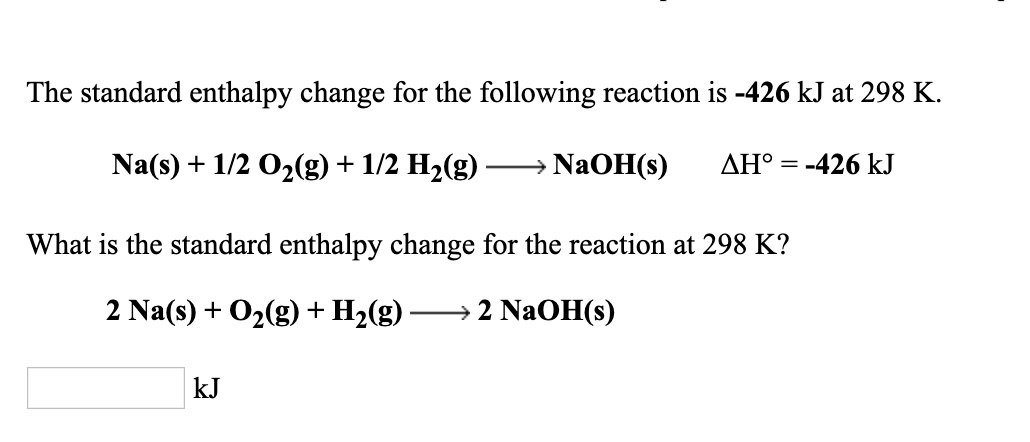The standard enthalpy change for the following reaction is -426 kJ at 298 K. Na(s) + 1/2 O2(g) + 1/2 H2(g) → NaOH(s) AH° = -426 kJ What is the standard enthalpy change for the reaction at 298 K? 2 Na(s) + O2(0)+ H2()—2 NaOH(s) The standard enthalpy change for the following reaction is 484 kJ at 298 K. CH3COOH(1) ► 2 C(s,graphite) + 2 H2(g) + O2(g) AH° = 484 kJ What is the standard enthalpy change for this...

• ### The standard enthalpy change for the following reaction is -774 kJ at 298 K. Fe(s) +...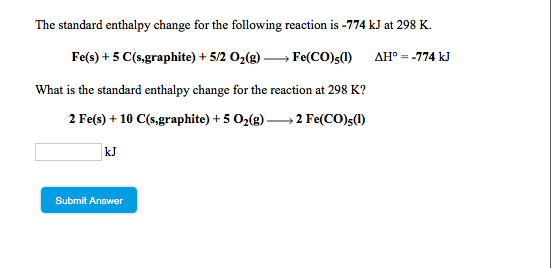The standard enthalpy change for the following reaction is -774 kJ at 298 K. Fe(s) + 5 C(s.graphite) + 5/2 O2(g) Fe(CO)(1) AH--774 kJ What is the standard enthalpy change for the reaction at 298 K? 2 Fe(s) + 10 C(s.graphite) +5 O2(g) → 2 Fe(CO)s(1) Submit Answer

• ### The standard enthalpy change for the following reaction is - 52.3 kJ at 298 K. C2H4(g)...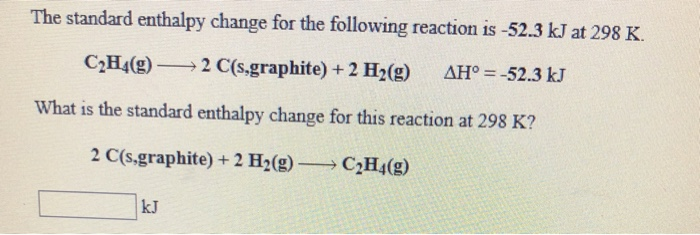The standard enthalpy change for the following reaction is - 52.3 kJ at 298 K. C2H4(g) — 2 C(s.graphite) + 2 H2(g) AH° = -52.3 kJ What is the standard enthalpy change for this reaction at 298 K? 2 C(s,graphite) + 2 H2(g) — C2H4(9) LLUCU The standard enthalpy change for the following reaction is -240 kJ at 298 K. Ni(s) + 1/2 O2(g) → Nio(s) AH° = -240 kJ What is the standard enthalpy change for the reaction at...

• ### The standard enthalpy change for the following reaction is -971 kJ at 298 K What is...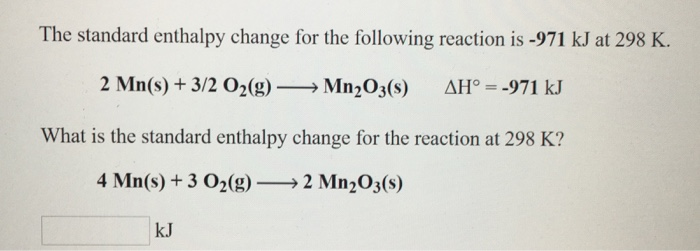The standard enthalpy change for the following reaction is -971 kJ at 298 K What is the standard enthalpy change for the reaction at 298 K? 4 Mn(s) + 3。2(g)-→ 2 Mn2O3(s) kJ

• ### The standard enthalpy change for the following reaction is -415 kJ at 298 K. Zn(s) + Ch(g) → ZnCl2() AH° = -415 k...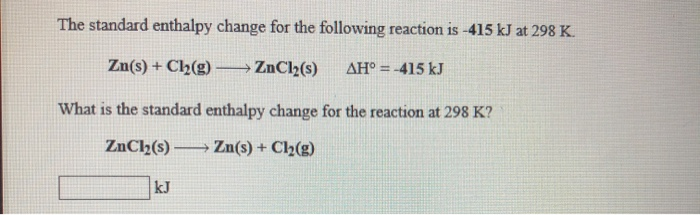The standard enthalpy change for the following reaction is -415 kJ at 298 K. Zn(s) + Ch(g) → ZnCl2() AH° = -415 kJ What is the standard enthalpy change for the reaction at 298 K? ZnCl(s) — Zn(s) + Cl2(g) The standard enthalpy change for the following reaction is -50.6 kJ at 298 K. N2H40 - N2(g) + 2 H2(g) AH° = -50.6 kJ What is the standard enthalpy change for this reaction at 298 K? N2(g) + 2 H2(g)...